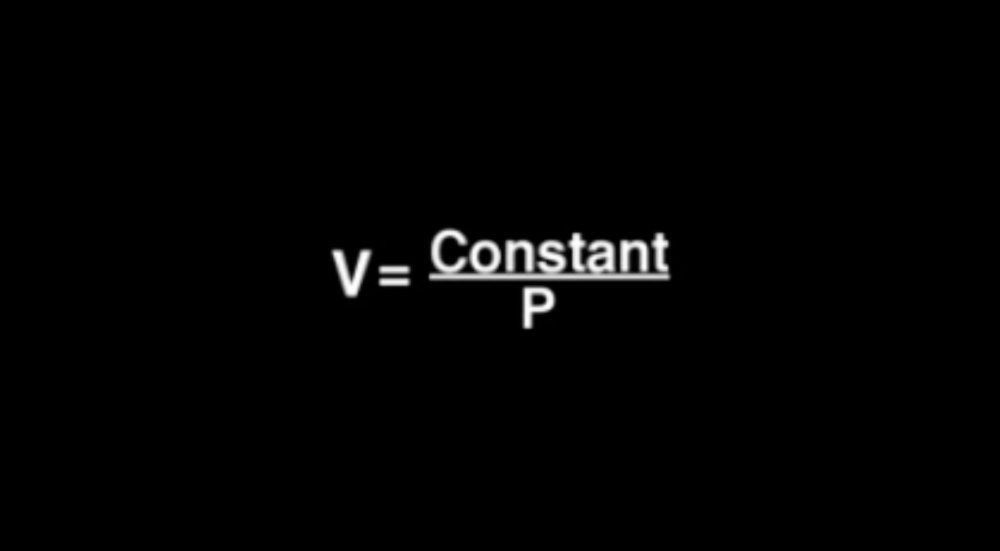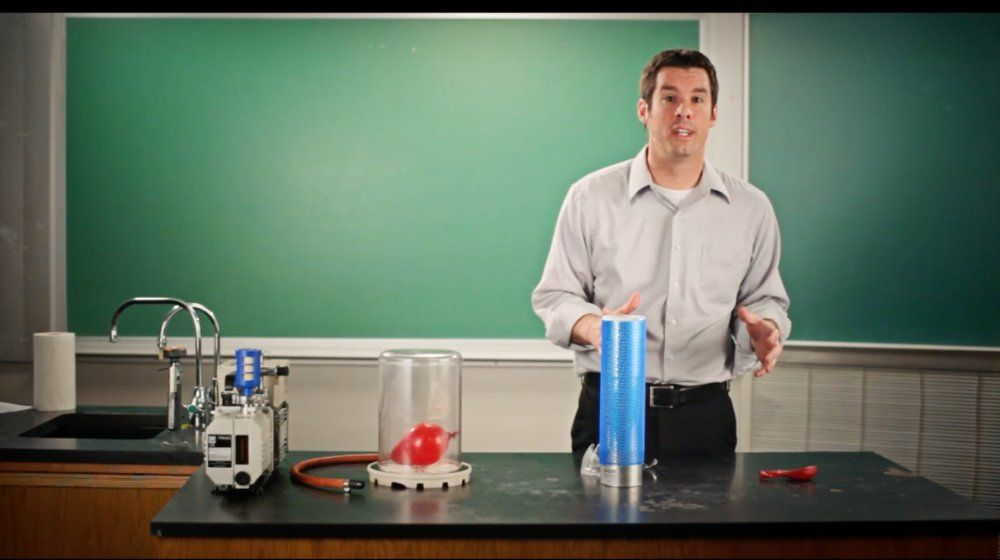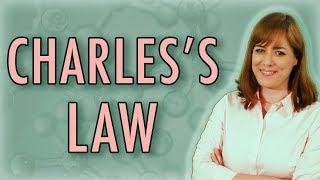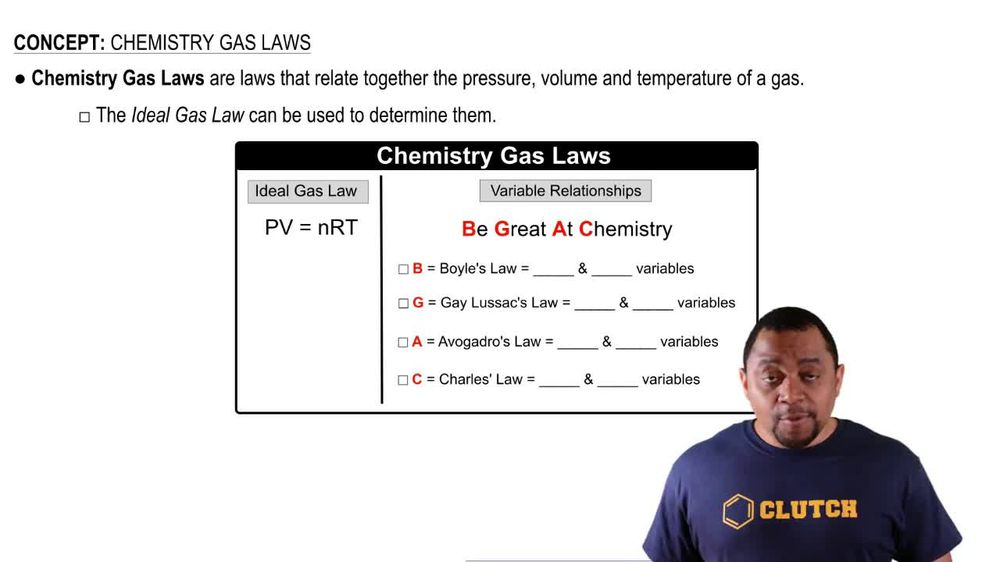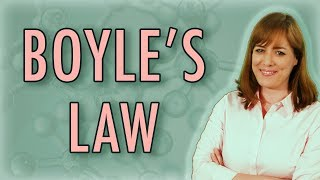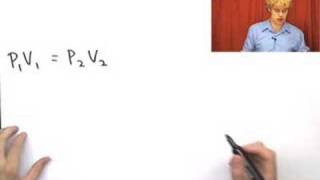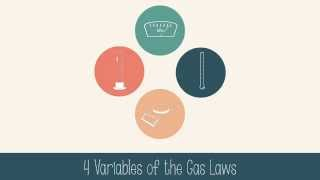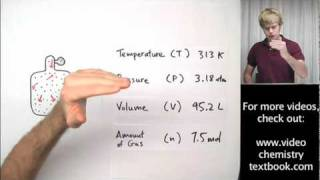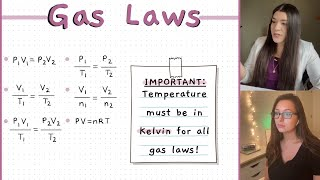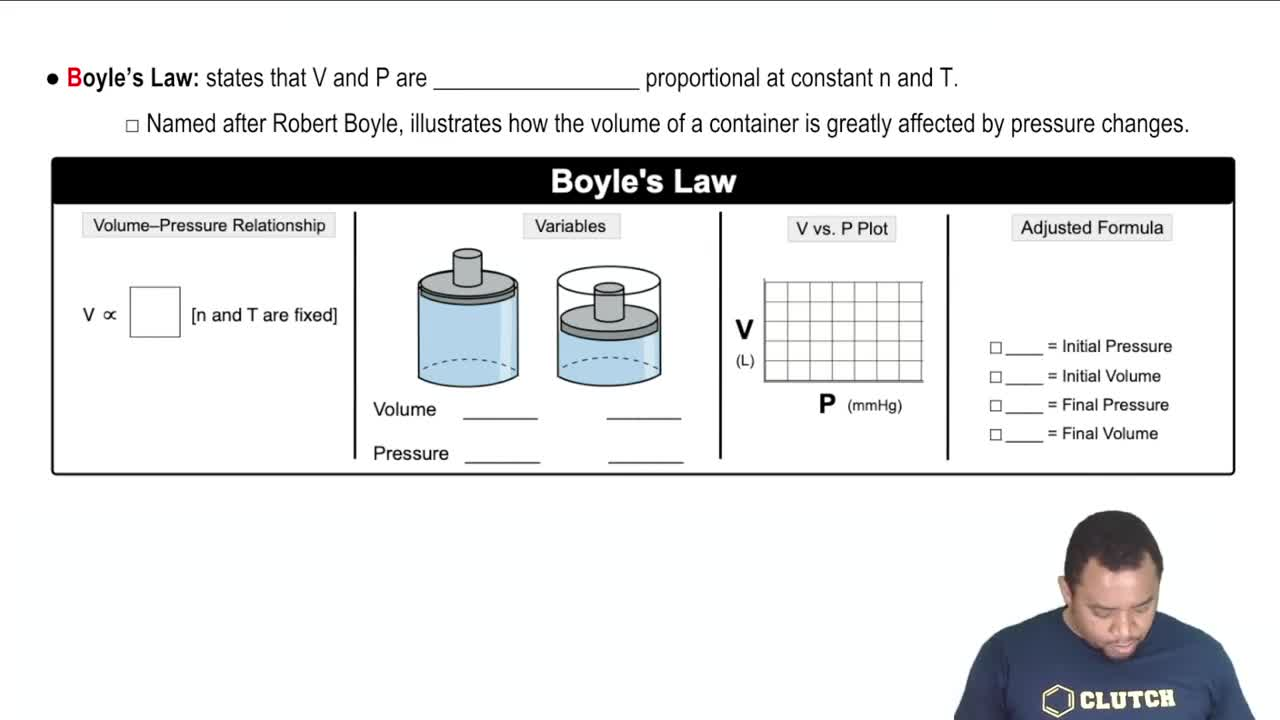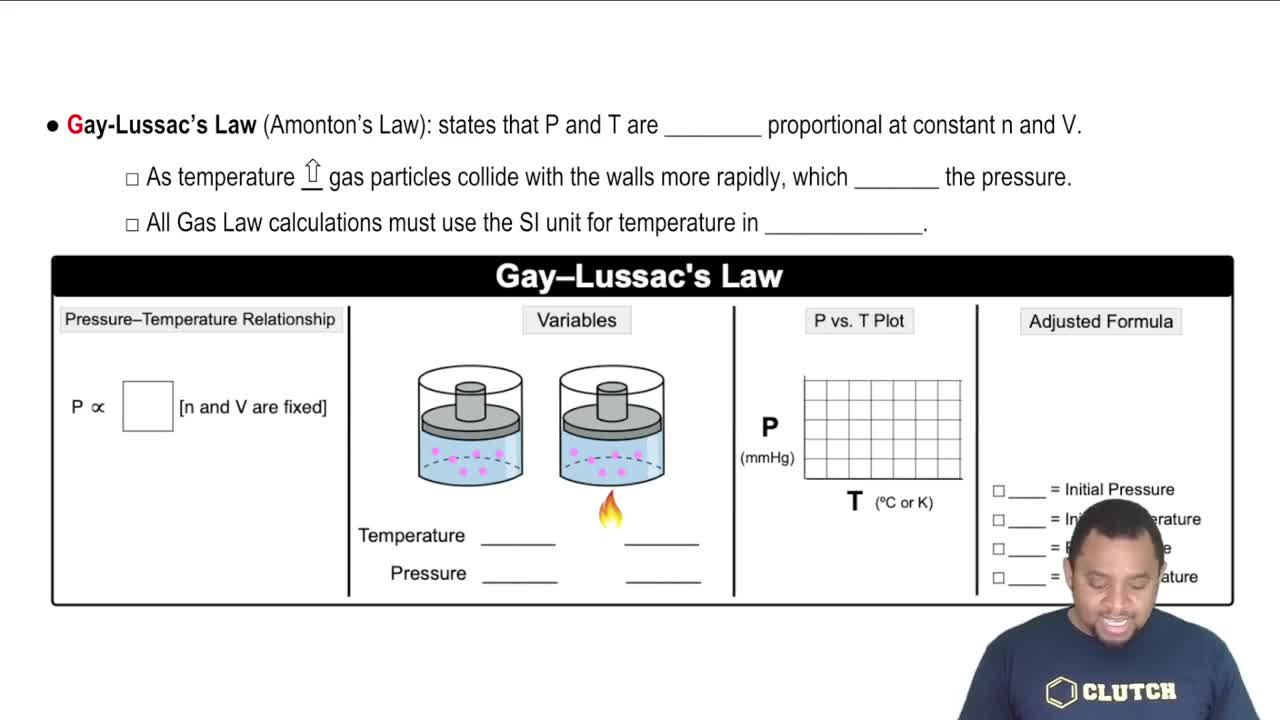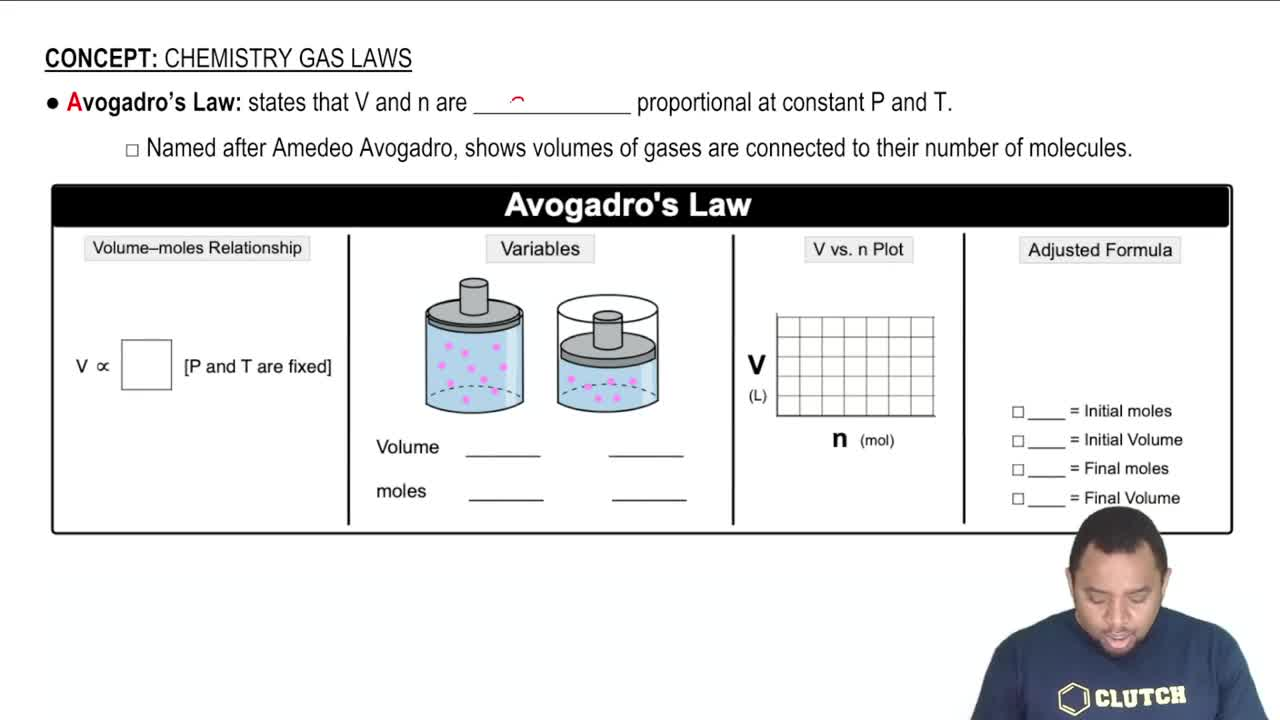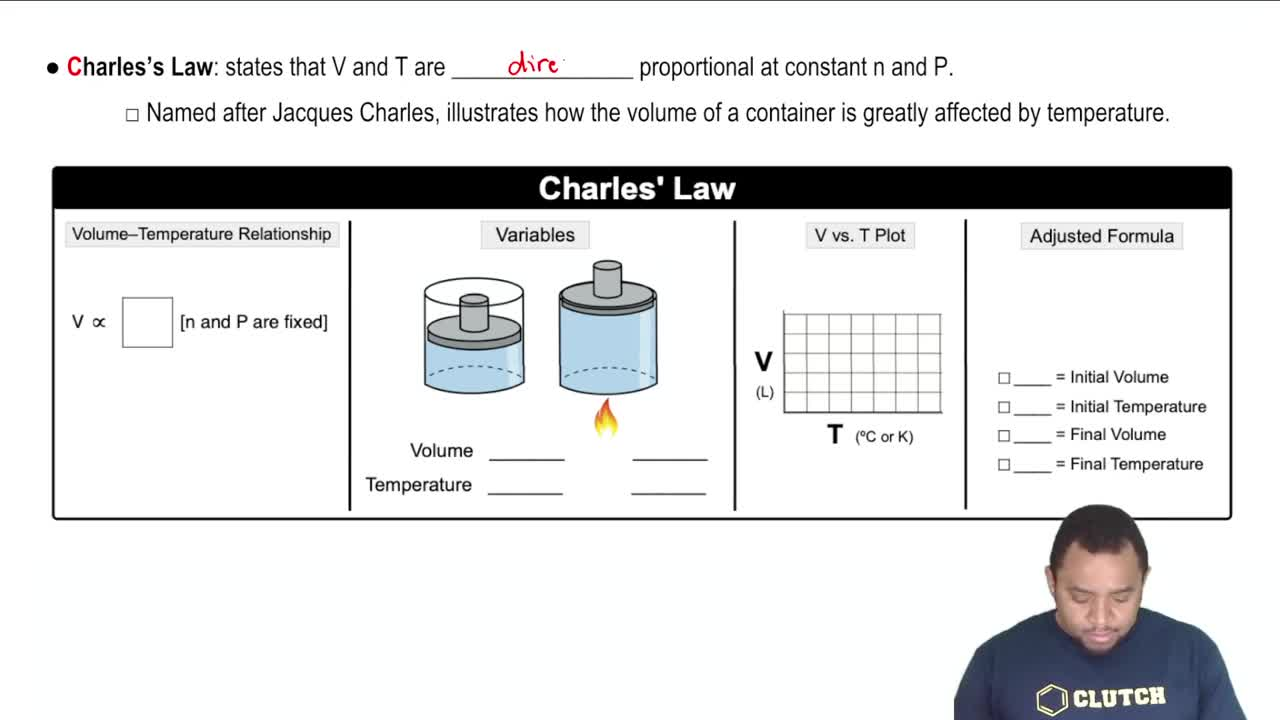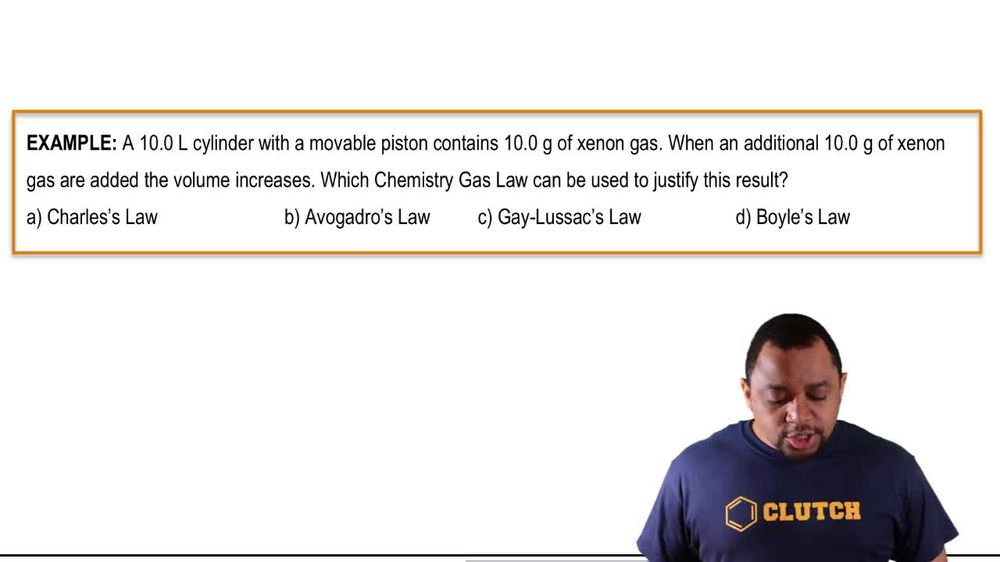Start typing, then use the up and down arrows to select an option from the list.
1. 7. Gases2. Chemistry Gas Laws# Chemistry Gas Laws

by Jules Bruno
71 views
0
oh God! Rose Law states that are volume V and our moles and are directly proportional at constant pressure, P and temperature T. Now it's named after Amadeo of um and it shows that the volumes of gasses are connected to their number of molecules here. We're going to say that the relationship between volume and moles is that V is directly proportional to moles. And again that happens when P and T are constant or fixed. The way we depict this with our mobile pistons is if we take a look here at this image, we're gonna say, this container has a lot of dots, so it has a lot of mold or number of molecules. So moles would be high to house all of these molecules. We'd want our volume to be high because gas is like it when there's an opt amount of distance between them. But what happens if I take some of these molecules of gasses out, what are moles of gas would be below and I no longer need as much space for them. So my volume would be low. They both are high together or low together when pressure and temperature are constant or fixed. Now, how do we depict this in a plot? Since they're directly proportional, you'd say this line of V. N. N. Which showed increasing over time, Where you could start out at 0l and it increases over time as our moles increase. Now, what would our adjusted formula or our avocados law formula B. That would just be the one over N one Equals V two over and two. So our initial variables here. Initial modes would be N one initial volume. V one. Final M.nolds will be in two. Final volume would be V two. So just remember our volume and moles are directly proportional, meaning they're both high together or low together when our pressure and temperature are constant or fixed.